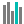#T.INV.2T (PQL - xl)

This function returns the t-value of the student's t-distribution as a function of the probability and the degrees of freedom

• Library: PQL \ Spreadsheet \ Statistical
• Compatibility: Any content (regardless of data source) in the Tabulate spreadsheet module

#### Syntax

T.INV.2T(probability, deg freedom)

##### Function Arguments
 Name Description Type Optional probability Probability of the two-tailed student’s t-distribution Number deg freedom Number of degrees of freedom to characterize the distribution; if not an integer, the number is truncated Number